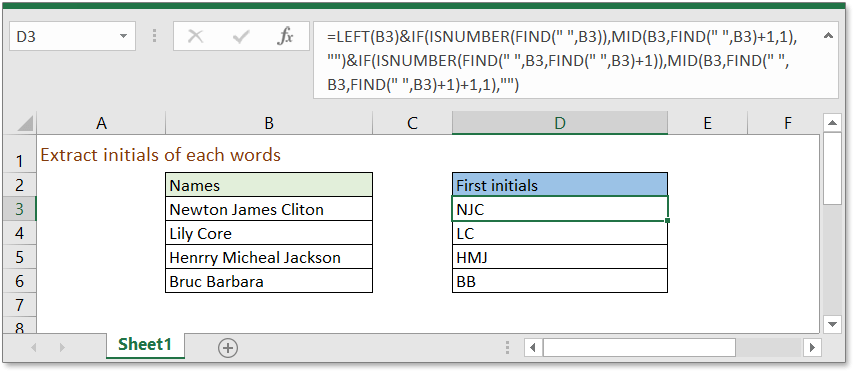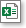## Excel Formula: Extract initials from names

There are several methods can extract each initials from a list of names in Excel, here in this tutorial, it provides a formula to handle this job.Generic formula:

 =LEFT(name)&IF(ISNUMBER(FIND(" ",name)),MID(name,FIND(" ",name)+1,1),"")&IF(ISNUMBER(FIND(" ",name,FIND(" ",name)+1)),MID(name,FIND(" ",name,FIND(" ",name)+1)+1,1),"")

Arguments

 Name: the full names you want to extract the initials.

Remark

This formula only extract initials from first three words, start from the forth word, it will ignore.

How this formula work

To extract initials from cell B3, please us below formula:

 =LEFT(B3)&IF(ISNUMBER(FIND(" ",B3)),MID(B3,FIND(" ",B3)+1,1),"")&IF(ISNUMBER(FIND(" ",B3,FIND(" ",B3)+1)),MID(B3,FIND(" ",B3,FIND(" ",B3)+1)+1,1),"")

Press Enter key, then the first letter of each word has been extracted.

Explanation

As this formula combine three formulas with “&”, here explains three formulas in three parts

First part LEFT(B3)

LEFT function extracts text from left side of a text based on the given position. Here LEFT(B3) extracts first letter from left side of the text in cell B3, “N”.

Second part IF(ISNUMBER(FIND(" ",B3)),MID(B3,FIND(" ",B3)+1,1),"")

FIND function finds a string within another string, and returns the starting position of the string inside another oneMID function returns the substring of a given text based on the given position and text length.

MID(B3,FIND(" ",B3)+1,1) returns
MID(B3,7+1,1) returns
J

ISNUMBER function returns TRUE if the cell contains number, if not, it returns FALSE.

IF function tests for a certain condition.

IF(ISNUMBER(FIND(" ",B3)),MID(B3,FIND(" ",B3)+1,1),"") returns
IF(ISNUMBER(7),”J”,"") returns
IF(TRUE,”J”,"") returns
J

Third part IF(ISNUMBER(FIND(" ",B3,FIND(" ",B3)+1)),MID(B3,FIND(" ",B3,FIND(" ",B3)+1)+1,1),"")

MID function extract text from a text based on the given position and number of length.

MID(B3,FIND(" ",B3,FIND(" ",B3)+1)+1,1) returns
MID(B3,FIND(" ",B3,7+1)+1,1) returns
MID(B3,13+1,1) returns
C

IF(ISNUMBER(FIND(" ",B3,FIND(" ",B3)+1)),MID(B3,FIND(" ",B3,FIND(" ",B3)+1)+1,1),"") returns
=IF(ISNUMBER(FIND(" ",B3,FIND(" ",B3)+1)),"C","") returns
=IF(ISNUMBER(13),"C","") returns
C

### Sample FileClick to download sample file

#### Relative Functions

• LEFT function
Extract text from left side.
• FIND function
find a string within another string, and returns the starting position of the string inside another one.
• IF function
Test for conditions.
• MID function
extract part of text from a cell value based on the location and length.

### The Best Office Productivity Tools

#### Kutools for Excel - Helps You To Stand Out From Crowd

 Popular Features: Find, Highlight or Identify Duplicates  |  Delete Blank Rows  |  Combine Columns or Cells without Losing Data  |  Round without Formula ... Super VLookup: Multiple Criteria  |  Multiple Value  |  Across Multi-Sheets  |  Fuzzy Lookup... Adv. Drop-down List: Easy Drop Down List  |  Dependent Drop Down List  |  Multi-select Drop Down List... Column Manager: Add a Specific Number of Columns  |  Move Columns  |  Toggle Visibility Status of Hidden Columns  |  Compare Columns to Select Same & Different Cells ... Featured Features: Grid Focus  |  Design View  |  Big Formula Bar  |  Workbook & Sheet Manager | Resource Library (Auto Text)  |  Date Picker  |  Combine Worksheets  |  Encrypt/Decrypt Cells  |  Send Emails by List  |  Super Filter  |  Special Filter (filter bold/italic/strikethrough...) ... Top 15 Toolsets:  12 Text Tools (Add Text, Remove Characters ...)  |  50+ Chart Types (Gantt Chart ...)  |  40+ Practical Formulas (Calculate age based on birthday ...)  |  19 Insertion Tools (Insert QR Code, Insert Picture from Path ...)  |  12 Conversion Tools (Numbers to Words, Currency Conversion ...)  |  7 Merge & Split Tools (Advanced Combine Rows, Split Excel Cells ...)  |  ... and more

Kutools for Excel Boasts Over 300 Features, Ensuring That What You Need is Just A Click Away...#### Office Tab - Enable Tabbed Reading and Editing in Microsoft Office (include Excel)

• One second to switch between dozens of open documents!
• Reduce hundreds of mouse clicks for you every day, say goodbye to mouse hand.
• Increases your productivity by 50% when viewing and editing multiple documents.
• Brings Efficient Tabs to Office (include Excel), Just Like Chrome, Edge and Firefox.